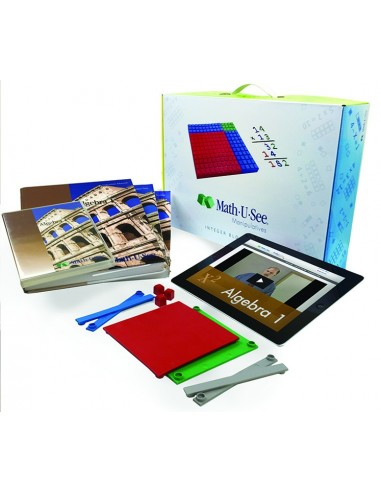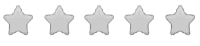See inside this book

# Math-U-See Algebra 1 Universal Set

\$182.00
No tax
Demme Learning
New Items
P-MA09-60Leave a Review

#### Description

New! Math-U-See Algebra 1 Universal Set
Math-U-See is a complete, skill-based, multi-sensory homeschool math curriculum for grades K-12 that's designed to teach students skills in a logical sequence.

The Algebra 1 Universal Set includes everything you need for a successful experience with Algebra 1: Instruction Manual with complete solutions, Instruction DVD, Student Workbook, Tests booklet, Integer Block Kit, Algebra/Decimal Insert Kit and 12 months of access to our new Algebra 1 Digital Pack.

Math-U-See Algebra covers Graphing, Simultaneous Equations, Exponents, Polynomials, Unit Multipliers, and more.

Table of Contents includes: Commutative and Associative Properties Order of Operations Solving for an Unknown with One Variable Distributive Property Cartesian Coordinates Graphing a Line Slope-Intercept Formula Graphing Parallel Lines and the Equation of a Line Graphing Perpendicular Lines Finding the Slope-Intercept Formula with Different Givens Graphing Inequalities Solving Simultaneous Equations by Graphing Solving Simultaneous Equations by Substitution Solving Simultaneous Equations by Elimination Coin Problems Consecutive Integers Multiplication and Division with Exponents Negative Exponents and Raising Exponents to a Power Addition and Multiplication of Polynomials Factor Polynomials Factor Trinomials with Coefficients Factor Trinomials with Negative Numbers Square Roots and Dividing Polynomials Difference of Two Squares and Oriental Squares Repeated Factoring of Polynomials Solving Equations with Factoring Unit Multipliers Square Unit Multipliers Metric Conversions Fractional Exponents Significant Digits and Scientific Notation Bases Other Than Ten Graphing a Circle and an Ellipse Graphing a Parabola and a Hyperbola